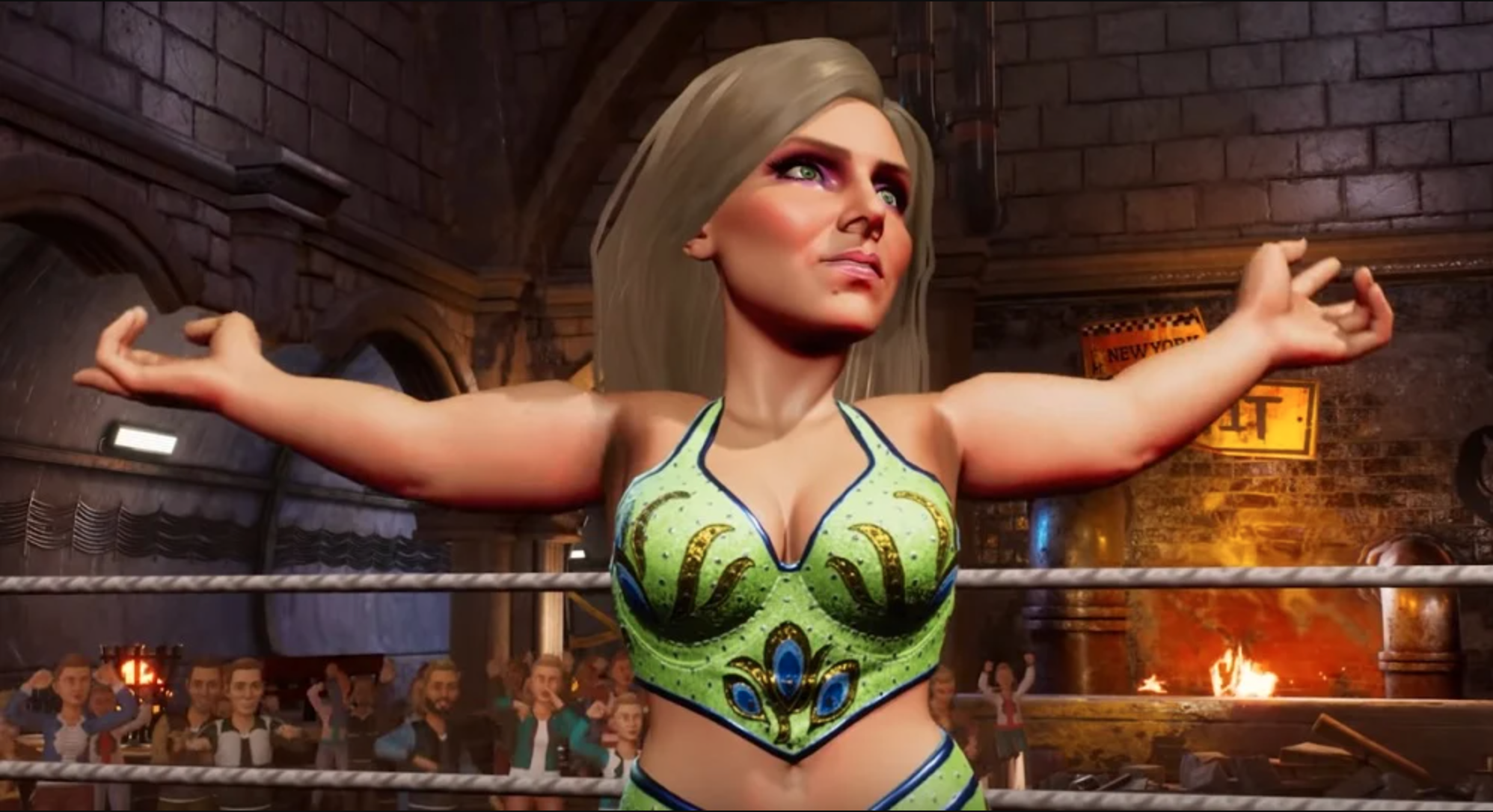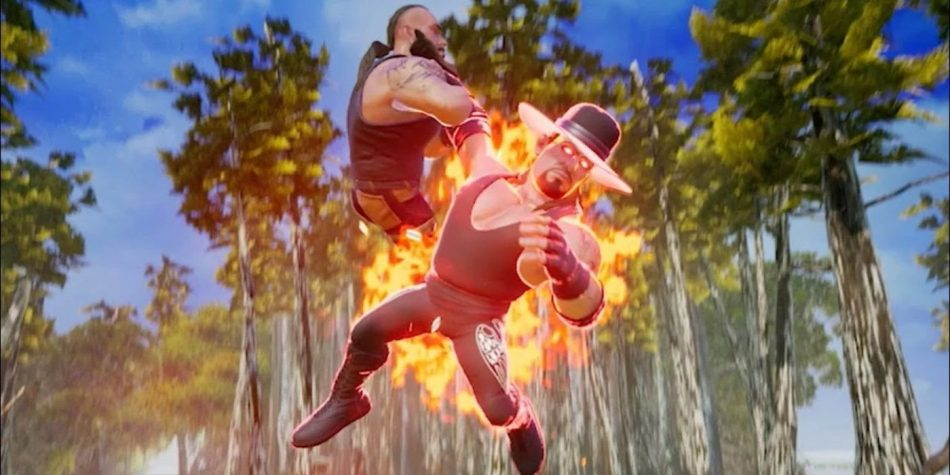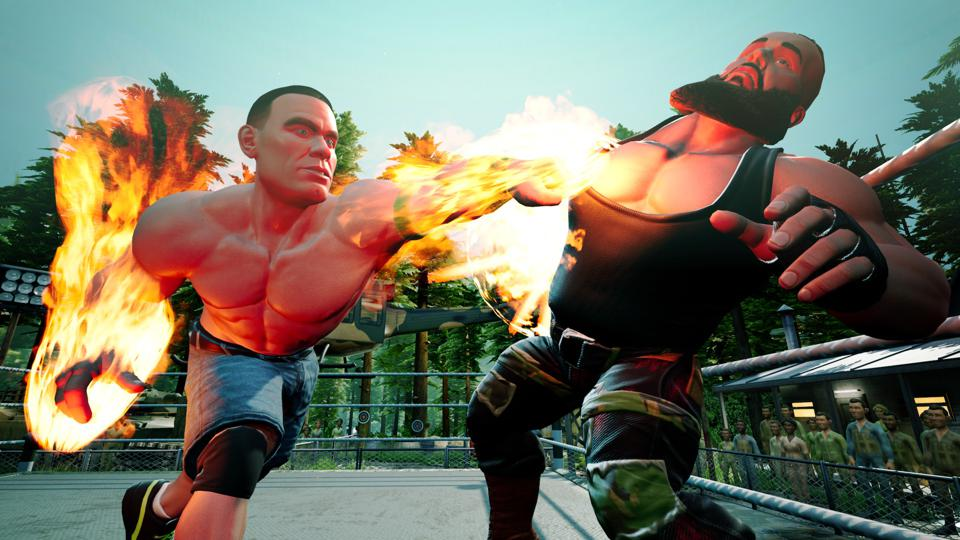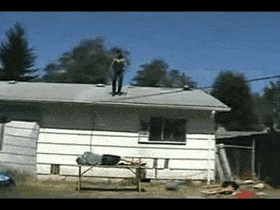WWE 2K Battlegrounds lets you suit up as one of your favorite WWE Superstars and take on your opponents and reign supreme as world champion.

Here, we're breaking down the WWE 2K Battlegrounds controls so you have a basic idea of how the game plays. It does not look like you can alter your controls as of the game’s launch, so get used to these controls.

Once you’ve got the basics down, check out our guide to really get a head lock on all your friends.

Basic MovesMove XBox One PlayStation 4 Nintendo Switch GrappleCarry (hold)Submission (near grounded opponent) A X B Pin (Near grounded opponent)Irish Whip (Near standing opponent) B Circle A KickSpringboard (running toward ropes) Y Triangle X PunchDive (running at ropes) X Square Y BlockParry (when being hit) RB R1 R Run (hold + direction) RT R2 ZR Combo Modifier LT L2 ZL Pick up objectClimb turnbuckleGo to apron LB L1 L Ask for help (tag team only) Left stick click L3 Left stick click Power-ups 1/2/3 + Taunt D-Pad Left/Up/Right/Down D-Pad Left/Up/Right/Down D-Pad Left/Up/Right/Down Target Different Opponent Special Throw Right stick click R3 Right stick click

In addition to the basic moves, each Superstar has a set of special moves that are unique to their archetype.

Special MovesHigh-Flyer Special Moves

 Move XBox One PlayStation 4 Nintendo Switch Forked Lightning Kick LT+A L2+X ZL+B Knee-High LT+Y L2+Triangle ZL+X Whiplash LT+X L2+Square ZL+Y

Powerhouse Special Moves

 Move XBox One PlayStation 4 Nintendo Switch Seismic Smash LT+A L2+X ZL+B Bigfoot LT+Y L2+Triangle ZL+X Driving Range LT+X L2+Square ZL+Y

Brawler Special Moves

 Move XBox One PlayStation 4 Nintendo Switch The Kitchen Sink LT+A L2+X ZL+B Feet First LT+Y L2+Triangle ZL+X Deepfake LT+X L2+Square ZL+Y

All-Around Special Moves

 Move XBox One PlayStation 4 Nintendo Switch Shooting Star LT+A L2+X ZL+B Reboot LT+Y L2+Triangle ZL+X Chin Up LT+X L2+Square ZL+Y Hit the Floor LT+Y (after Irish Whip) L2+Triangle (after Irish Whip) ZL+X (after Irish Whip)

Technician Special Moves

 Move XBox One PlayStation 4 Nintendo Switch Power Surge LT+A L2+X ZL+B Fist of the Dragon LT+X L2+Square ZL+Y

Further still, each archetype has their own punch and kick combos that you’ll need to learn.

Don’t worry – they’re simple!

CombosHigh-Flyer Combos

 Move XBox One PlayStation 4 Nintendo Switch Roundhouse Ear Ringer X, Y, Y Square, Triangle, Triangle Y, X, X Rotisserie Kickin' Y, X, Y Triangle, Square, Triangle X, Y, X Cyclonic Kick Y, Y, Y Triangle, Triangle, Triangle X, X, X Spin Cycle X, X, X Square, Square, Square Y, Y, Y

Powerhouse Combos

 Move XBox One PlayStation 4 Nintendo Switch Coffin Nail X, X, X Square, Square, Square Y, Y, Y Chopping Block X, Y, X Square, Triangle, Square Y, X, Y Earmuffs Y, X, X Triangle, Square, Square X, Y, Y Stone Crusher Y, Y, Y Triangle, Triangle, Triangle X, X, X

Brawler Combos

 Move XBox One PlayStation 4 Nintendo Switch Bull Rush X, X, X Square, Square, Square Y, Y, Y Jewel Thief X, Y, X Square, Triangle, Square Y, X, Y Glasgow Kiss Y, X, X Triangle, Square, Triangle X, Y, Y Vicious Cycle Y, Y, Y Triangle, Triangle, Triangle X, X, X

All-Around Combos

 Move XBox One PlayStation 4 Nintendo Switch Overhead Charge Y, X, X Triangle, Square, Square X, Y, Y Toothache X, Y, X Square, Triangle, Square Y, X, Y Morning Star X, X, X Square, Square, Square Y, Y, Y Flying Face Lift Y, Y, Y Triangle, Triangle, Triangle X, X, X Wildfire Whip X, Y, Y Square, Triangle, Triangle Y, X, X Blazing Big Boot Y, X, Y Triangle, Square, Triangle X, Y, X

Technician Combos

 Move XBox One PlayStation 4 Nintendo Switch Pistol Whip X, X, X Square, Square, Square Y, Y, Y Boot Sandwich Y, Y, Y Triangle, Triangle, Triangle X, X, X Falling Star X, Y, Y Square, Triangle, Triangle Y, X, X Thunderclap Y, X, Y Triangle, Square, Triangle X, Y, X

Now get in the ring and get to work, greenhorn! (Or check out our tips and tricks guide to if you don't wanna get your ass whooped.)For more quick gaming guides, check out or Gaming page.

For gaming jokes, memes, and inappropriate videos, check out our Gaming Facebook page.